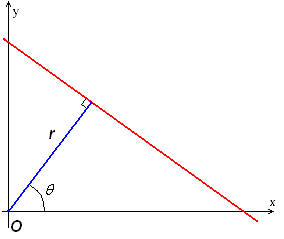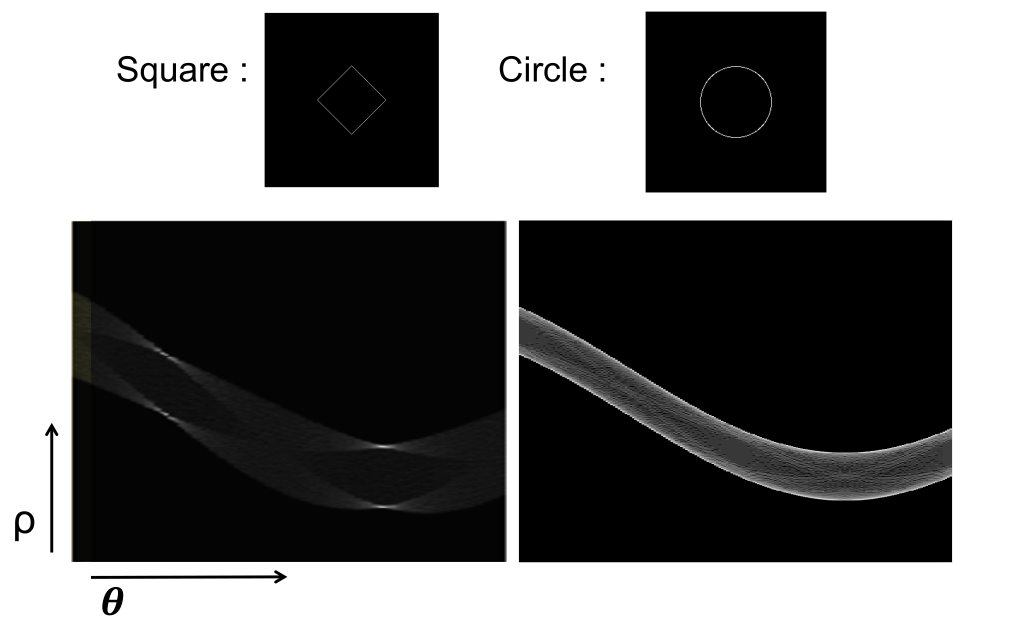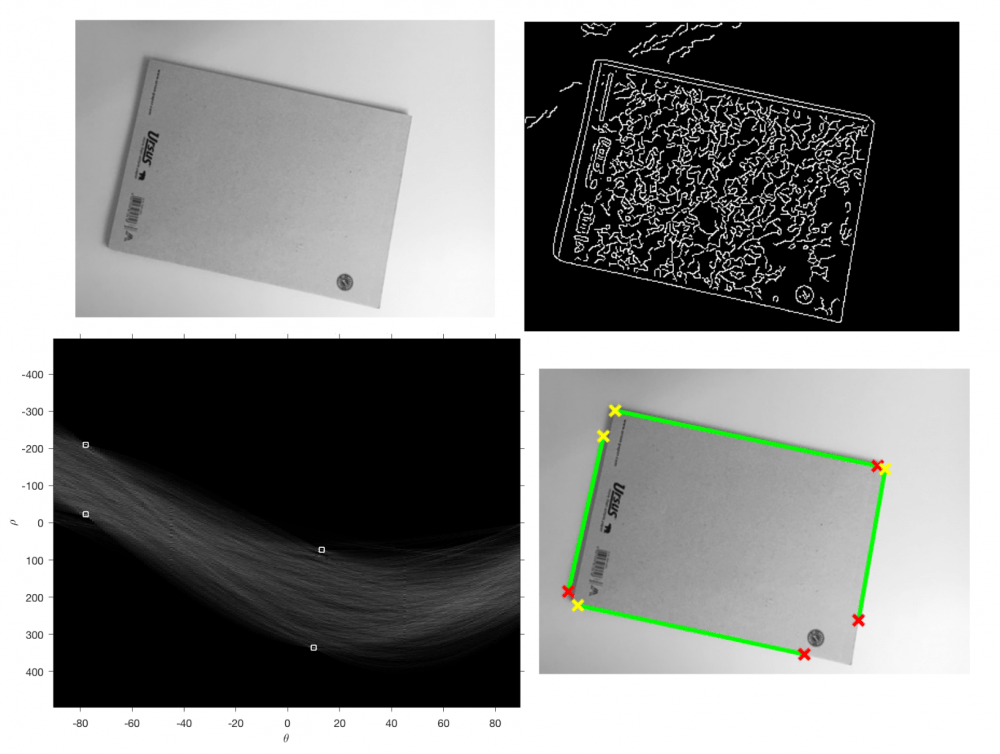# The Hough transform

In image analysis, we often may want to detect lines in an image. This is useful for many things, including segmentation, tracking, etc.
A classic edge detector is the Canny edge detector. Below you can see an example of the Canny edge detector applied to a photograph of a steam engine (source: Wikipedia).This is great, but it doesn’t tell us any lines – just which pixels are in an edge or not. Typically, when we think of a mathematical line, the equation
$$y = mx + b$$
comes to mind. How can we get a set of $(m,b)$ parameters from our Canny-filtered image? This is what the Hough transform is for.

## Parametrizing lines

A line on a plane can be described using two parameters. The typical parametrization is $y = mx + b$, where the two parameters are $m$ and $b$. This does however have some issues: the slope of a vertical line would be infinite. A better choice of parameters is polar parameters, $(r, \theta)$.We still use two parameters, just now we use the distance from the origin, $r \ge 0$, and the angle $\theta \in [0, 2\pi]$ of the normal connecting the line to the origin. So for vertical lines we simply have $\theta = 0$ or $\theta = \pi$. If we work out the trigonometry, the equation for our line becomes:

$$x\cos\theta + y\sin\theta = r.$$

## The Hough space

Now that we have a parametrization we can image plotting $r$ against $\theta$ in a graph. This plane we are working in is sometimes called Hough space. A line in the Cartesian (or image) plane would correspond to a point in Hough space – a single $(r,\theta)$ pair.
If we took the set of all lines passing through a point on the Cartesian plane, what would it look like in Hough space? If we fix $x = x_0$ and $y = y_0$, we get

$$r(\theta) = x_0\cos\theta + y_0\sin\theta,$$

which means we’re looking at a sinusoidal.

So, lines become points and points become sinusoidals.

## The Hough transform

The idea of the Hough transform is to count how many edge pixels in our image lie on every possible line, or $(r,\theta)$ pair. Then we can find the $(r, \theta)$ pairs with the most edge pixels on them – and voilà, we have our line parameters.
Now of course, we can’t really check every possible $(r,\theta)$ pair. Instead we will discretize them into bins. For instance we could say we will increment $\theta$ in intervals of $1$ degree and $r$ in increments of one, from $0$ to, say, $100$. This would give us $360 \cdot 100 = 36\,000$ bins.
Now we can run the following algorithm:

1. Initialize an empty $(n_\theta \times n_r)$ array of bins
2. For every edge pixel $(x_0, y_0)$:
• For $\theta = 0 … 360$ in intervals of $\frac{360}{n_\theta}$:
1. Calculate $r = x_0\cos\theta + y_0\sin\theta$
2. Increment bin $(\lfloor \frac{r}{r_{max}} \cdot n_r \rfloor,\theta)$ by one
3. Find the bins with the most “votes”. The corresponding $(r,\theta)$ are the main lines in the image.

This is a very broad definition of the algorithm: there is a lot to be said about the number of bins we should use, how we should select the “most voted” bins, and the effect these things have on the result – but the general idea is there.

### Examples

Here are a couple of examples taken from my Image Analysis and Computer Vision course at ETH.In this image we can see what the result of the algorithm is in Hough-space. On the left we have a square which, of course, has four flat sides. We can clearly see there are four “hotspots” in the Hough-transformed image.
On the other hand, a circle has no straight edges, so there is no clear “brightest spot” in the Hough transform.Here we see one very bright spot – this corresponds to the longest edge in the picture, the upper edge of the larger block.Finally, the full process: from the original image, we apply some edge detector (typically Canny), and run the Hough transform. Here one further step is shown: by looking at which edge pixels actually lie on the line, we can define segments in the image – this is another process worthy of a much more in-depth look.

## Final remarks

The Hough transform is a very effective method for finding lines in images, but, as always, it has its limitations. It is quite computationally intensive, and noise in the edges can cause it to “detect” multiple lines that are close to each other instead of just one line. This can be mitigated in many ways: using fewer bins and smoothing the Hough-transformed image before detecting peaks are two examples, both with drawbacks.
Peak detection itself is a tricky business: a simple threshold often just won’t cut it. One way to improve the robustness of the peak detection would be to use the direction and magnitude of the gradient of the original image to apply “weights” to the detected lines.
After that, the process of creating segments needs to be refined: edges output from the Canny edge detector are often noisy or interrupted, leading to the formation of multiple segments which could be connected into one.